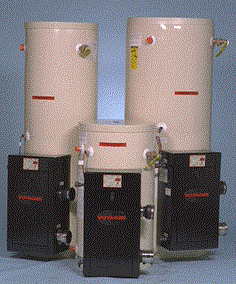A# water heater energy efficiencyBefore you purchase a water heater for your home, it's important to know how energy efficient it is. Usually the most energy-efficient water heater models or systems are more expensive. However, if you purchase an energy-efficient model, you may save more money in the long run on your water heating bills than if you purchase a less efficient model.

## Energy efficiency of storage, demand, and heat pump water heaters

Use the energy factor to determine the energy efficiency of a storage, demand, or heat pump water heater.

The energy factor (EF) indicates a water heater's overall energy efficiency based on the amount of hot water produced per unit of fuel consumed over a typical day. This includes the following:

• Recovery efficiency – how efficiently the heat from the energy source is transferred to the water
• Standby losses – the percentage of heat loss per hour from the stored water compared to the heat content of the water (water heaters with storage tanks)
• Cycling losses – the loss of heat as the water circulates through a water heater tank, and/or inlet and outlet pipes.
•

The higher the energy factor, the more efficient the water heater. However, higher energy factor values don't always mean lower annual operating costs, especially when you compare fuel sources.

When choosing a storage, demand, or heat pump water heater model, you need to factor in not only energy efficiency but alsio:

• fuel type and availability
• size
• costs
•

## Solar water heater energy efficiency

For a solar water heating system, use the solar energy factor (SEF) and solar fraction (SF) to determine its energy efficiency.

The solar energy factor is defined as the energy delivered by the system divided by the electrical or gas energy put into the system. The higher the number, the more energy efficient. Solar energy factors range from 1.0 to 11. Systems with solar energy factors of 2 or 3 are the most common.

Another solar water heater performance metric is the solar fraction. The solar fraction is the portion of the total conventional hot water heating load (delivered energy and tank standby losses). The higher the solar fraction, the greater the solar contribution to water heating, which reduces the energy required by the backup water heater. The solar fraction varies from 0 to 1.0. Typical solar factors are 0.5–0.75.

Don't choose a solar water heating system based solely on its energy efficiency. When selecting a solar water heater, it's also important to consider the following:

• size
• overall cost